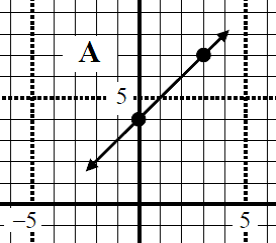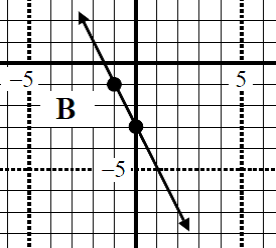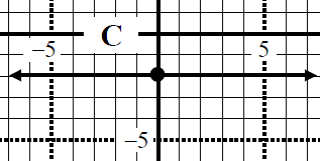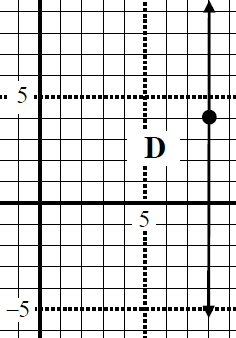# Perpendicular line equation#### Everything You Need in One Place

Homework problems? Exam preparation? Trying to grasp a concept or just brushing up the basics? Our extensive help & practice library have got you covered.#### Learn and Practice With Ease

Our proven video lessons ease you through problems quickly, and you get tonnes of friendly practice on questions that trip students up on tests and finals.#### Instant and Unlimited Help

Our personalized learning platform enables you to instantly find the exact walkthrough to your specific type of question. Activate unlimited help now!##### Intros
###### Lessons
1. How to find the equation of a perpendicular line?
##### Examples
###### Lessons
1. Given the graph of linear equation, find the slope of perpendicular line equation.

1.2.3.4.2. The lines 3y + 7x = 3 and cy - 2x - 1 = 0 are perpendicular. Find "c"
1. Determine the equation of a line that is perpendicular to the line 3y + 5x = 8, and passes through the origin. Answer in slope intercept form and general form.
###### Topic Notes
In this lesson, we will look at questions related to perpendicular line equation. We will try to determine perpendicular line equation with different given information such as, graphs, equations of other lines and points.